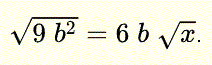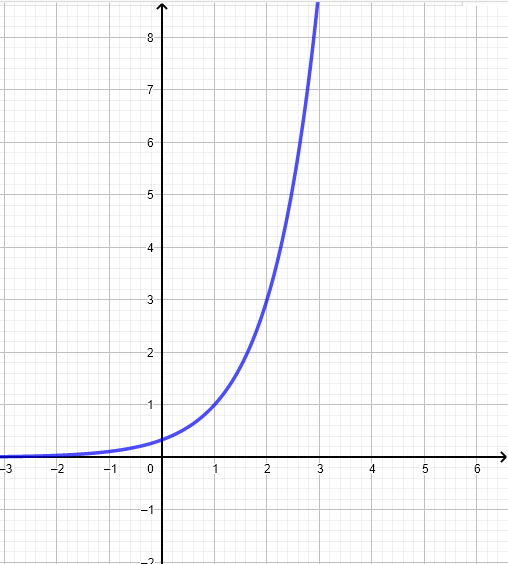# Grade 11 Math Practice Test Questions

Grade 11 math practice test questions are presented along with their solutions on videos.

1. Simplify the following expressionsSolution on video at Simplify Square Root Expressions question 1

2. Simplify the following expressionSolution on video at Simplify Square Root Expressions Using the Conjugate question 2

3. Expand and simplify the following expressionSolution on video at Expand and Simplify Polynomials , question 3

4. b and x are positive real numbers such thatFind x .
Solution on video at Solve Equations with Square Roots , question 4


5. Simplify and express as a single rational expression (2 parts a and b)
a) $$\quad \displaystyle \frac{2x}{x-1}\:-\:\frac{1}{x+2}-\frac{6}{2x^2+2x-4}$$.
Solution on video at Add Rational Expressions and Simplify, question 5 a

b) $$\quad \displaystyle \frac{2x^2+2x-4}{x^2+8x+15} \div \frac{2x^2+6x+4}{x^2+10x+21}$$.
Solution on video at Divide Rational Expressions and Simplify , question 5 b

6. Simplify the expression and write the result with positive exponents only.
$$\quad \displaystyle \frac{(3 \: x^2 y^2)^2}{(- 2 \: x y^2)^4} \:\div \:\frac{(3 \: x y)^3}{(6 \: x^{-1} y^2)^2}$$.
Solution on video at Simplify Rational Expression with Expoenents, question 6

7. Solve the quadratic inequality $$-x^2-2x \gt - 2$$
Solution on video at solve quadratic inequalities, question 7

8. Let $$f(x) = - x^2 + 2 x + 2$$.
a) Find the vertex of the graph of $$f$$
b) Find the x and y intercepts of the graph of $$f$$
c) Find the equation of the axis of symmetery of the graph of $$f$$
d) What are the domain and range of $$f$$ ?
d) Graph $$f$$.

9. a) Find the equation of the quadratic function $$f$$ whose graph is shown below and write it in the form $$f(x) = a x^2 + b x + c$$.
b) Find the exact values of the x intercept of the graph of $$f$$.10. Find the equation of the exponential function of the form $$g(x) = a^{x-b}$$, where $$a$$ and $$b$$ are constants to be found, and whose graph is shown below.11. Solve the equation
$$\displaystyle \frac{2x+1}{x-2}\:=-1\:-\:\frac{1}{x+1}$$.

12. Use special angles and trigonometric formulas to find the exact value of
a)   $$\displaystyle \cos (75^{\circ} )$$         b)   $$\displaystyle \sec (15^{\circ} )$$

13. Find the exact value of
a)   $$\displaystyle \tan (-330^{\circ} )$$         b)   $$\displaystyle \csc (480^{\circ} )$$

14. Prove the idendity
a)   $$\displaystyle \cot x + \sec x \sin x = 1+\tan x$$

15. Find angle $$\theta$$ in the range $$[0 , 360^{\circ} )$$ such that
a)   $$\displaystyle \tan( \theta ) = 0.2$$         b)   $$\displaystyle \cos(\theta + 30^{\circ} ) = 0.5$$

16. The water depth $$d$$ (in meters) in a harbor at $$t$$ hours after midnight is given by $$d(t) = 7.2 \cos ( 30^{\circ}(t - 6.5) ) + 5.8$$
a) What is the maximum depth of water and when does it occur?
b) What is the minimum depth of water and when does it occur?
c) Sketch $$d$$ as a function of $$t$$ over two periods.

17. Sketch the graph of $$y = - 2^{x-2} - 3$$

18. Sketch the graph of $$y = -3 \cos (x - 30^{\circ}) + 3$$

19. Simplify the expression
$$\displaystyle \left( 2^{\frac{1}{5}} x^{\frac{1}{2}} \right) \left( 16^{\frac{1}{5}} x^{\frac{1}{2}} \right)$$.

20. Solve the equation
$$\displaystyle \frac{1}{8 ^x \; 4^x}\:= 2^{-7x+\frac{1}{2}}$$.

21. Find all values of $$m$$ so that the equation in $$x$$ given below has two real solutions.
$$\displaystyle 2x^2 - x + m = 1$$.

22. Find the points of intersection of the graph of the following pair of equations.
$$\displaystyle (x - 2)^2 + (y + 1)^2 = 5$$ and $$\displaystyle 4x - 2 y = 4$$ .

23. The third term of a geometric sequence is equal to $$-18$$ and the fourth term is equal to $$54$$. Find the seventh term of the sequence and the sum of the first ten terms of the sequence.

24. What amount must be invested in order to have $$\20 000$$ in $$10$$ years at the rate of $$6\%$$ compunded semi annually ?